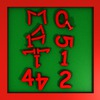## Math Minute - EquationsSolving the X-files was easier then finding the solution of some equations in a minutes!

## Math Minute AnywhereA Math Minute game that you can play anywhere, anytime.

## Math Minute - SequencesGuessing the next number in a sequence is a hard logical task, and you have only a minute to solve as many as you can.

## Gallows-treeSlow paced animated hangman game that features thousands of English words to guess.

## Math MinuteMath Minute is a short, fast paced game that improves your head numeracy skills with arithmetic puzzles.

## Math QuizFifteen puzzles where you should find the odd one out of four mathematical operations that gives a different outcome.

## Math Minute - CoinsThe second installment of the successful Math Minute series teaches you how can be summed the coins in your pocket under a minute.

## LogiminoA puzzle game with shapes that are composed of five squares. They are seems too easy at first but just few percent of player could have solved all of the puzzles.